# Investors’ Hope Brightened, as Wema Bank Maintained Positive Retained Earnings-Q3-2018Coy: Wema Bank Plc

Current Market Price: N0.63

NPL RATIIO:

Year High: N1.54

Year Low: N 0.50

Fair Value: N2.07

By: Jeariogbe Tunde Segun (Equity Analyst)

Key Financial Ratios

• This report observed the nine months financial performance of Wema Bank Plc for the period ended 30th September, 2018, and compare same with figures in similar quarter of 2017
• It was observed that Wema Bank Retained Earnings is now positive to the tune of N7.544 billion as at the end of the third quarter of the year. This has further increased investors’ hope of receiving some rewards at the end of the ongoing financial year.
• We also established a positive performance in both top and bottom line of Wema Bank financial statistics for the period ended 30th September, 2018.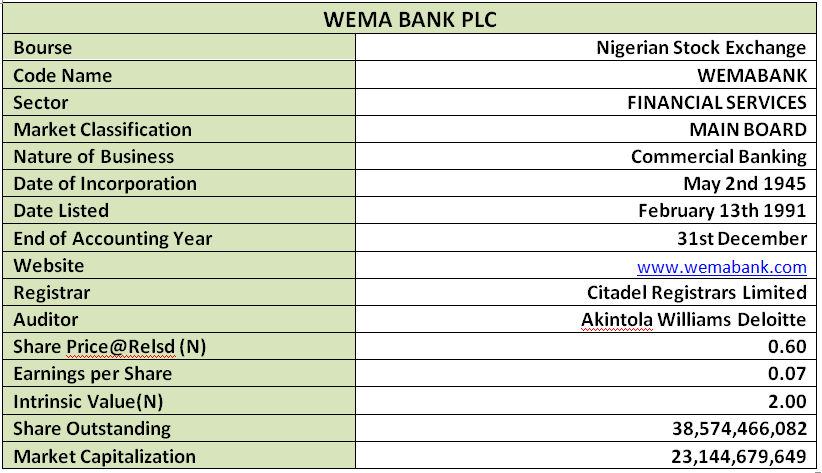Company Figures

• Gross earnings for the period only improved by 7.51% above what was reported in 2017 Q3. A total of N43.498 billion was reported for the nine months as against N45.565 billion
• Interest Income stood at 3.77% above 2017 figure. Current Interest Income is N38.888 billion as against the N37.473 billion posted in 2017 nine months result
• Meanwhile, Interest Expenses dropped by 8.64%, having moved from the previous N25.242 billion to N23.061 billion
• Profit before Tax currently reported is N3.056 billion, this is 69.87% above  the N1.799 of 2017
• Having considered other costs including tax expense, N2.644billion was reported as the Profit for the period. The said figure is 78.88% above the N1.529 billion that was reported in corresponding quarter in 2017
• Total comprehensive income improved by 74.09% having moved to N2.662 billion as against N1.529 billion in the previous third quarter
• Total Assets was currently estimated at N480.771 billion, same as 21.88% above the N394.459 billion estimated at the end of 2017 third quarter financial performance
• Total Liabilities is currently N428.789 billion, that is 24.48% above the N344.459 billion reported in corresponding quarter of 2017
• Total Deposit through the nine months currently reported is estimated at N374.778 billion, this is 27.17%% above the N294.702 billion achieved in the first nine months of 2017
• Meanwhile, unlike other financial institutions, Loans and Advances improved qoq by 16.09%. According to the report a total of N244.790 billion was given out as loans and advances through the period this is above the N210.866 billion of 2017
• Net Assets achieved marginal growth to the tune of 3.96% when compared to the reported figure in similar period of 2017.
• Kindly see the table below for details.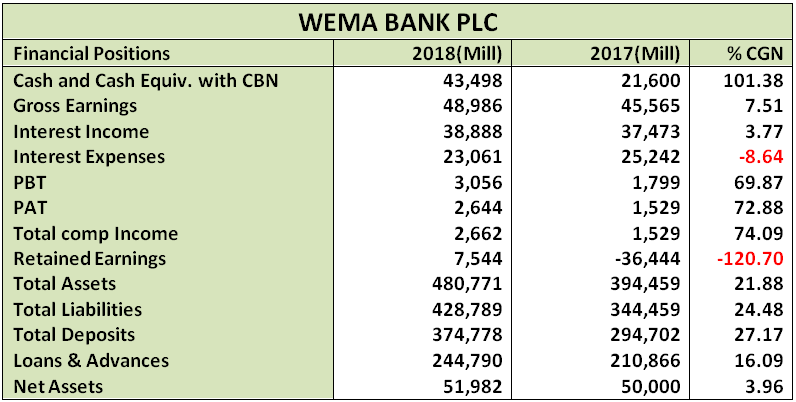Volatility Ratios

• Going by the estimated beta value of Wema Bank Plc, the share is currently enjoying heightened patronage as it currently runs a beta value higher than unity and above industrial average.
• Although almost irrelevant since we analyze a financial institution whose major business is to collect deposit (mostly reported under liability) we estimated Debt to Equity ratio as 53.57% below the industrial average of 64.44%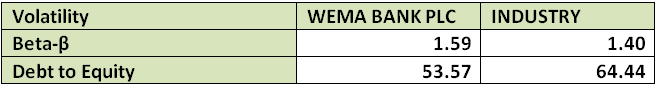Profitability Ratios

• Interest Expense to Gross Earnings is presently estimated at 47.08% this is 15.02% below the 55.40% estimated in 2017 nine months financial statistics
• PBT margin stood at 6.24% as against 3.95% last year, this is 58.01% improvement
• In our opinion, Profit Margin is currently poor when compared to other financial institution’s estimates. We have estimated 5.40% margin from Gross Earnings as against the previous estimate of 3.36%
• Return on Average Equity is now 5.09% compared to the 3.06% returns achieved in the first nine months of 2017
• Return on Average Assets only differs by 41.85%%, moving from 0.39% to 0.55%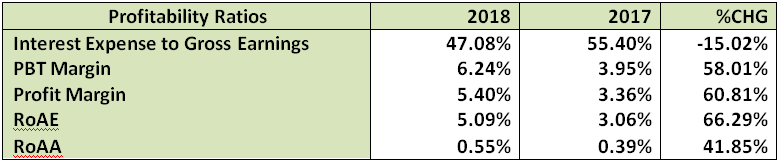Efficiency Ratio

• Gross Earnings to Total Assets is estimated at 10.19% this is 11.79% below the estimate in 2017
• Meanwhile, Gross Earnings to Equity is now 92.24% as against the 91.13% estimated from 2017 nine months financial statistics
• Financial Leverage is 9.25x as against 7.89x, this is an estimate of the number of times the total assets replicates the equity, in other words the ratio gets better
• It was also established that 63.52% of the Total Deposit was given out as Loan and Advances during the period under estimate this is 8.72% lower than the 71.55% given out during the first nine months of 2017
• Meanwhile, Loan and Advances is same as 50.92% of the Total Assets, this is 4.75% lower than the 53.46% of last nine months financial activities.Investment Ratios

• The estimated amount earned per units of Wema Bank shares is N0.07, this is 72.88% above the N0.04 earned in 2017 Q3 business period.
• The said earnings per share yielded 11.42% of the current market price on the day the result was made available to the investing public. This is a better yield when compared to the 7.93% achieved the last Q3
• PE/Ratio for the period is 2.92x as against 4.20x estimated last year.
• As we speak the Book Value of Wema Bank is N1.35 fairly same as N1.30 last year. Should we choose to value this equity with this indices, we shall conclude an underpriced position of each units of its shares on the floor of the exchange
• Further confirming the underpriced state of Wema Bank Shares is the Price to Book Value ratio that stood below unity.
• Opex Margin was grew by 15.31% having moved to 41.73% from 36.19%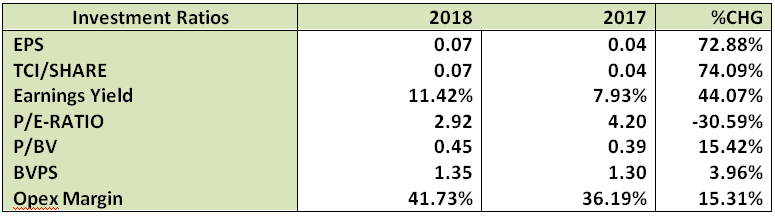Valuation

Through our blend of valuation model, fully considering the non dividend state of the financial institution over the period, we have valued each units of Wema Bank Plc at N2.07. Thus, considering the current market price of N0.63 (as at the time this report was concluded), we Rate Wema Bank shares a Buy.

# Investors’ Hope Brightened, as Wema Bank Maintained Positive Retained Earnings-Q3-2018Coy: Wema Bank Plc

Current Market Price: N0.63

NPL RATIIO:

Year High: N1.54

Year Low: N 0.50

Fair Value: N2.07

By: Jeariogbe Tunde Segun (Equity Analyst)

Key Financial Ratios

• This report observed the nine months financial performance of Wema Bank Plc for the period ended 30th September, 2018, and compare same with figures in similar quarter of 2017
• It was observed that Wema Bank Retained Earnings is now positive to the tune of N7.544 billion as at the end of the third quarter of the year. This has further increased investors’ hope of receiving some rewards at the end of the ongoing financial year.
• We also established a positive performance in both top and bottom line of Wema Bank financial statistics for the period ended 30th September, 2018.Company Figures

• Gross earnings for the period only improved by 7.51% above what was reported in 2017 Q3. A total of N43.498 billion was reported for the nine months as against N45.565 billion
• Interest Income stood at 3.77% above 2017 figure. Current Interest Income is N38.888 billion as against the N37.473 billion posted in 2017 nine months result
• Meanwhile, Interest Expenses dropped by 8.64%, having moved from the previous N25.242 billion to N23.061 billion
• Profit before Tax currently reported is N3.056 billion, this is 69.87% above  the N1.799 of 2017
• Having considered other costs including tax expense, N2.644billion was reported as the Profit for the period. The said figure is 78.88% above the N1.529 billion that was reported in corresponding quarter in 2017
• Total comprehensive income improved by 74.09% having moved to N2.662 billion as against N1.529 billion in the previous third quarter
• Total Assets was currently estimated at N480.771 billion, same as 21.88% above the N394.459 billion estimated at the end of 2017 third quarter financial performance
• Total Liabilities is currently N428.789 billion, that is 24.48% above the N344.459 billion reported in corresponding quarter of 2017
• Total Deposit through the nine months currently reported is estimated at N374.778 billion, this is 27.17%% above the N294.702 billion achieved in the first nine months of 2017
• Meanwhile, unlike other financial institutions, Loans and Advances improved qoq by 16.09%. According to the report a total of N244.790 billion was given out as loans and advances through the period this is above the N210.866 billion of 2017
• Net Assets achieved marginal growth to the tune of 3.96% when compared to the reported figure in similar period of 2017.
• Kindly see the table below for details.Volatility Ratios

• Going by the estimated beta value of Wema Bank Plc, the share is currently enjoying heightened patronage as it currently runs a beta value higher than unity and above industrial average.
• Although almost irrelevant since we analyze a financial institution whose major business is to collect deposit (mostly reported under liability) we estimated Debt to Equity ratio as 53.57% below the industrial average of 64.44%Profitability Ratios

• Interest Expense to Gross Earnings is presently estimated at 47.08% this is 15.02% below the 55.40% estimated in 2017 nine months financial statistics
• PBT margin stood at 6.24% as against 3.95% last year, this is 58.01% improvement
• In our opinion, Profit Margin is currently poor when compared to other financial institution’s estimates. We have estimated 5.40% margin from Gross Earnings as against the previous estimate of 3.36%
• Return on Average Equity is now 5.09% compared to the 3.06% returns achieved in the first nine months of 2017
• Return on Average Assets only differs by 41.85%%, moving from 0.39% to 0.55%Efficiency Ratio

• Gross Earnings to Total Assets is estimated at 10.19% this is 11.79% below the estimate in 2017
• Meanwhile, Gross Earnings to Equity is now 92.24% as against the 91.13% estimated from 2017 nine months financial statistics
• Financial Leverage is 9.25x as against 7.89x, this is an estimate of the number of times the total assets replicates the equity, in other words the ratio gets better
• It was also established that 63.52% of the Total Deposit was given out as Loan and Advances during the period under estimate this is 8.72% lower than the 71.55% given out during the first nine months of 2017
• Meanwhile, Loan and Advances is same as 50.92% of the Total Assets, this is 4.75% lower than the 53.46% of last nine months financial activities.Investment Ratios

• The estimated amount earned per units of Wema Bank shares is N0.07, this is 72.88% above the N0.04 earned in 2017 Q3 business period.
• The said earnings per share yielded 11.42% of the current market price on the day the result was made available to the investing public. This is a better yield when compared to the 7.93% achieved the last Q3
• PE/Ratio for the period is 2.92x as against 4.20x estimated last year.
• As we speak the Book Value of Wema Bank is N1.35 fairly same as N1.30 last year. Should we choose to value this equity with this indices, we shall conclude an underpriced position of each units of its shares on the floor of the exchange
• Further confirming the underpriced state of Wema Bank Shares is the Price to Book Value ratio that stood below unity.
• Opex Margin was grew by 15.31% having moved to 41.73% from 36.19%Valuation

Through our blend of valuation model, fully considering the non dividend state of the financial institution over the period, we have valued each units of Wema Bank Plc at N2.07. Thus, considering the current market price of N0.63 (as at the time this report was concluded), we Rate Wema Bank shares a Buy.